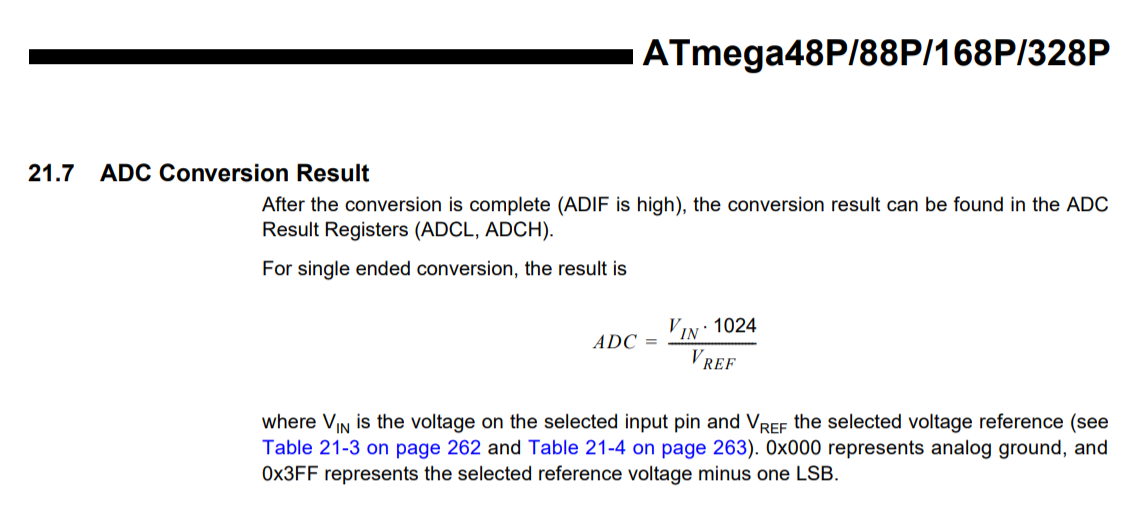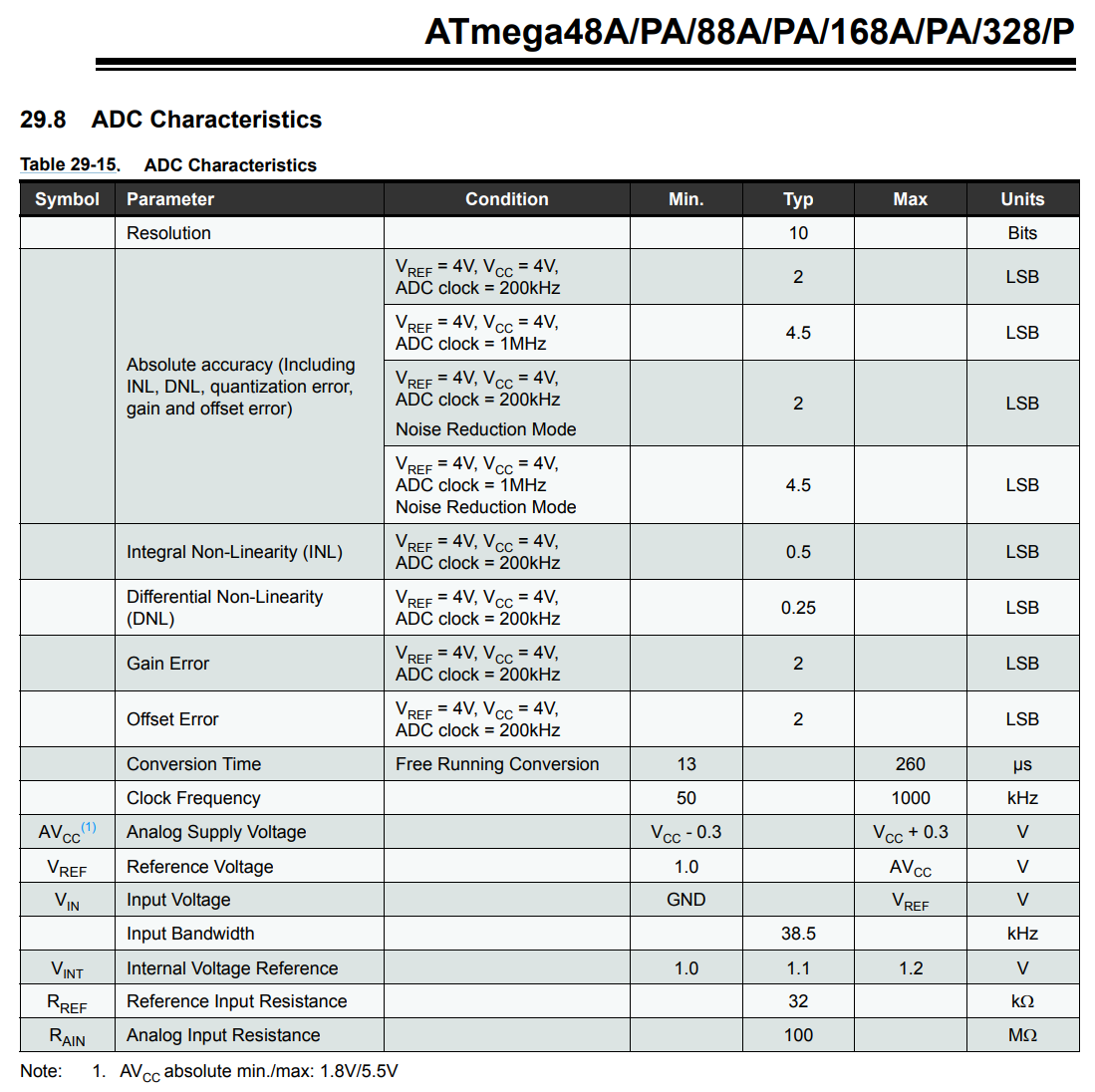# Inside Arduino

## How can I print multiple variables using Serial.println?

A common question in our courses and, indeed, online such as on Arduino StackExchange and the Arduino forums is some variation of: “How can I print multiple variables in one line of code using `Serial.println`?”

Here are some common answers. Note: I have not stress tested them all and I’m sure many solutions are slow and memory inefficient (but if neither of these are concerns, then feel free to use them!)

First, perhaps the simplest way is to cast everything as a String and use string concatenation:

``````Serial.println((String)"Var 1:" + var1 + " Var 2:" + var2 + " Var 3:" + var3);
``````

Source

Note: you should only do this for rapid prototypes because of memory inefficiencies with creating Strings in `C`; see “The Evils of Arduino Strings”

Second, you could use an external library such as PrintEx.

Third, you could redirect `printf` to Serial output:

## What’s calling loop() and how fast?

Because Arduino is open source, we can look up the source code to answer this question.

In short, `loop()` is called within an infinite `for` (or `while` loop). The only overhead is checking for whether there is data available on the serial port and then reading the serial buffers. The entire `int main(void)` function in main.cpp is:

Interestingly, this Arduino forum post suggests that because `serialEventRun()` is weakly defined in the core, you can define it locally in your sketch to override the default definition, which, according to the OP, will “save a little memory and makes the loop() run a little faster too!” You can do this if you don’t need to user serial communication.

To convert an `analogRead` value to voltage, should we divide by 1023 or 1024?

There is an interesting thread on Arduino forums discussing the merits of 1023 vs. 1024 as divisors. The maximum `analogRead` value is 1023; however, there are 1024 ‘steps’ between 0 and 5V. The official Arduino tutorial uses 1023—which effectively translates 0 - 1023 to 0 to 5V; however, others argue that this is wrong.

I think the key here is to remember that an ADC conversion represents a range of values with a step size of `5V/1024 = 0.0048828125V`. So if `analogRead` returns 0, this is really a range of 0V to 0.0048828125V, and 1 is a range of 0.0048828125V to 0.009765625V, etc. In that regard, we would want to divide analogRead by 1024 and if analogRead returns 1023, 1023/1024 * 5V = 4.9951171875V to 5V.

The ATmega datasheet says:For most practical purposes, dividing by 1023 or 1024 won’t matter. :)

For more on this hotly debated issue, read:

## What does delay() actually do?

As you might expect—given our warnings about avoiding overuse of `delay(int ms)`—the delay code consists of a `while` loop that simply waits for the given amount of delay time to pass. There is a `yield()` call within the `while` loop but this is, by default, an empty function—though you could implement it to create a “real cooperative scheduler.” The code for `yield()` is here.

The `delay(int ms)` function is found in wiring.c and is, in its entirety, copied below:

## How does the Arduino Uno ADC work exactly?

To convert analog signals to digital, the ATmega328 uses a successive approximation ADC, which Wikipedia nicely summarizes as: “a type of analog-to-digital converter that converts a continuous analog waveform into a discrete digital representation via a binary search through all possible quantization levels before finally converging upon a digital output for each conversion.”

In “Encyclopedia of Electronic Components Volume 3”, Platt states that a “successive approximation converter uses a single comparator, comparing the input voltage with the output from a DAC. The binary number that is supplied to the DAC is determined one bit at a time, from the most significant to the least significant bit, using the comparator’s result to determine if the bit should be 0 or 1. These bits are stored in a register, called a successive approximation register (SAR). When the process finishes, the SAR contains a binary representation of the input voltage. This type of ADC can achieve high resolution (many bits) at the cost of lower conversion speed.

The ATmega328 datasheet says that the analog input resistance is 100 megohms:• https://electronics.stackexchange.com/a/67173